# Stability of characteristic exponents

(diff) ← Older revision | Latest revision (diff) | Newer revision → (diff)

A property of the (Lyapunov) characteristic exponents (cf. Lyapunov characteristic exponent) of a linear system of ordinary differential equations(1)

where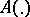is a continuous mapping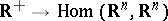(or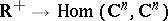), satisfying the condition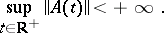One says that the characteristic exponents of the system (1) are stable if each of the functionsis continuous at the point. Hereare the characteristic exponents of the system (1) and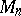is the set of all systems (1), equipped with the structure of a metric space given by the distance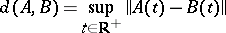(for convenience the system (1) is identified with the mapping; moreover, instead ofone writes).

Systems (1) with unstable exponents have been found (cf. , ). For example, the characteristic exponents of the system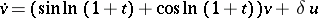for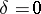are unstable, since forthe largest characteristic exponentis 1, and for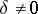,and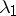does not depend on. For the stability of the characteristic exponents it is sufficient that the integral separation condition should be fulfilled (Perron's theorem). The set of systems (1) satisfying this condition coincides with the interior (in the space) of the set of all systems (1) with stable characteristic exponents.

Iffor allorfor all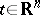(for a certain) (i.e. the system (1) has constant or periodic coefficients), then the characteristic exponents of the system (1) are stable. Ifis an almost-periodic mapping (cf. Linear system of differential equations with almost-periodic coefficients), then for the stability of the characteristic exponents of the system (1) it is necessary and sufficient that the system (1) be almost reducible (cf. also Reducible linear system).

For the characteristic exponents of the system (1) to be stable it is sufficient that there is a Lyapunov transformation reducing the system (1) to block-diagonal form: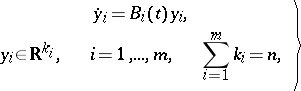(2)

such that: a) the blocks are integrally separable, i.e. numbers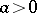,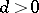can be found such that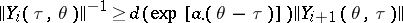for all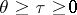,(here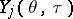is the Cauchy operator for the system (2)); and b) the upper and lower central exponents of the system (2) are equal to each other:The conditions of this theorem are also necessary for the stability of the characteristic exponents of the system (1) (cf. ). Systems with unstable characteristic exponents may possess the property of stochastic stability of the characteristic exponents.

The characteristic exponents of the system (1) are called stochastically stable (or almost-certainly stable) if for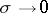the characteristic exponents of the system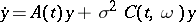tend with probability 1 to the characteristic exponents of the system (1); here the elements of the matrix giving the linear operator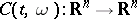(in a certain basis ofwhich is independent of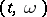) are independent non-null white noise.

If the mappingis uniformly continuous and ifthen for almost-every mapping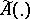, where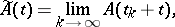the characteristic exponents of the systemare stochastically stable (for the shift dynamical system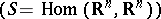one considers a normalized invariant measure, concentrated on the closure of the trajectory of the point; by "almost-every A" one means almost-everyin the sense of each such measure).

Let a dynamical system on a smooth closed manifoldbe given by a smooth vector field. Then for almost-every point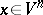(in the sense of each normalized invariant measure) the characteristic exponents of the system of variational equations associated with the trajectory of the pointare stochastically stable.

How to Cite This Entry:
Stability of characteristic exponents. Encyclopedia of Mathematics. URL: http://encyclopediaofmath.org/index.php?title=Stability_of_characteristic_exponents&oldid=18227
This article was adapted from an original article by V.M. Millionshchikov (originator), which appeared in Encyclopedia of Mathematics - ISBN 1402006098. See original article